# 4.5   Case Study: Small-World Phenomenon

The mathematical model that we use for studying the nature of pairwise connections among entities is known as the graph. Some graphs exhibit a specific property known as the small-world phenomenon. It is the basic idea that, even though each of us has relatively few acquaintances, there is a relatively short chain of acquaintances separating us from one another.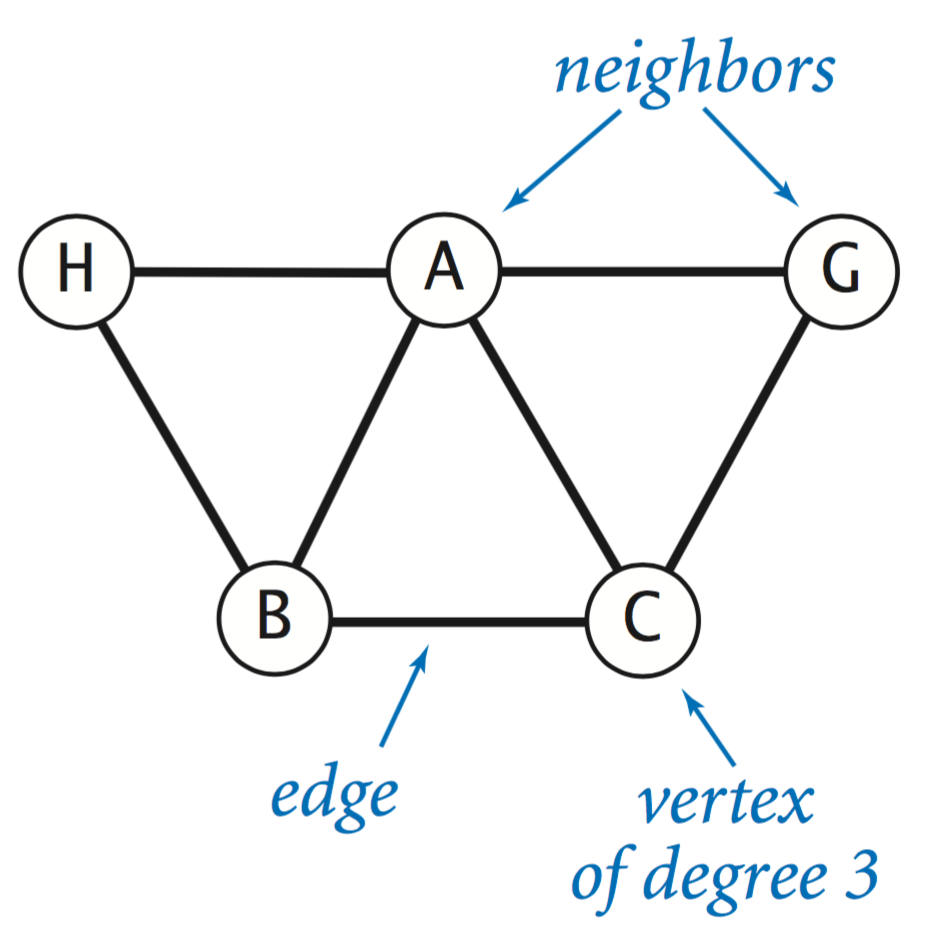## Graphs.

A graph is comprised of a set of vertices and a set of edges. Each edge represents a connection between two vertices. Two vertices are neighbors if they are connected by an edge, and the degree of a vertex is its number of neighbors.
The list below suggests the diverse range of systems where graphs are appropriate starting points for understanding structure.

GRAPH VERTICES EDGES
circulatory organ blood vessel
skeletal joint bone
nervous neuron synapse
social person relationship
epidemiological person infection
chemical molecule bond
n-body particle force
genetic gene mutation
biochemical protein interaction
Internet computer cable
distribution power station power line
mechanical joint beam
software module call
financial account transaction

## Graph data type.

Graph-processing algorithms generally first build an internal representation of a graph by adding edges, then process it by iterating over the vertices and over the vertices adjacent to a given vertex. The following API supports such processing:## Graph implementation.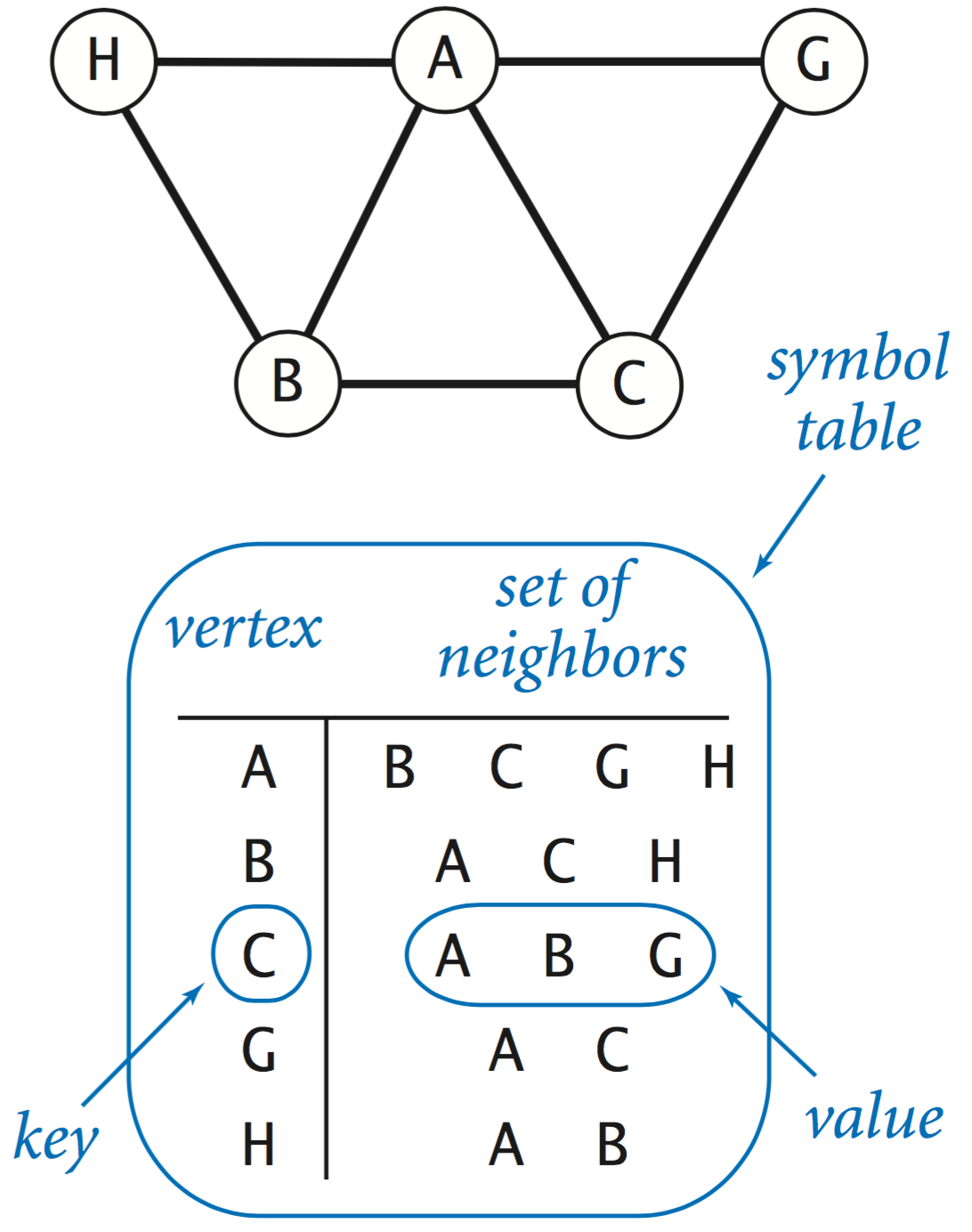Graph.java implements this API. Its internal representation is a symbol table of sets: the keys are vertices and the values are the sets of neighbors (the vertices adjacent to the key) This representation uses the two data types ST.java and SET.java.

Our implementation has three important properties:

• Clients can efficiently iterate through the graph vertices.
• Clients can efficiently iterate through a vertex's neighbors.
• Space usage is proportional to the number of edges.

## Performer–movie graphs.

The performer–movie graph includes a vertex for each performer and movie and an edge between a performer and a movie if the performer appeared in the movie.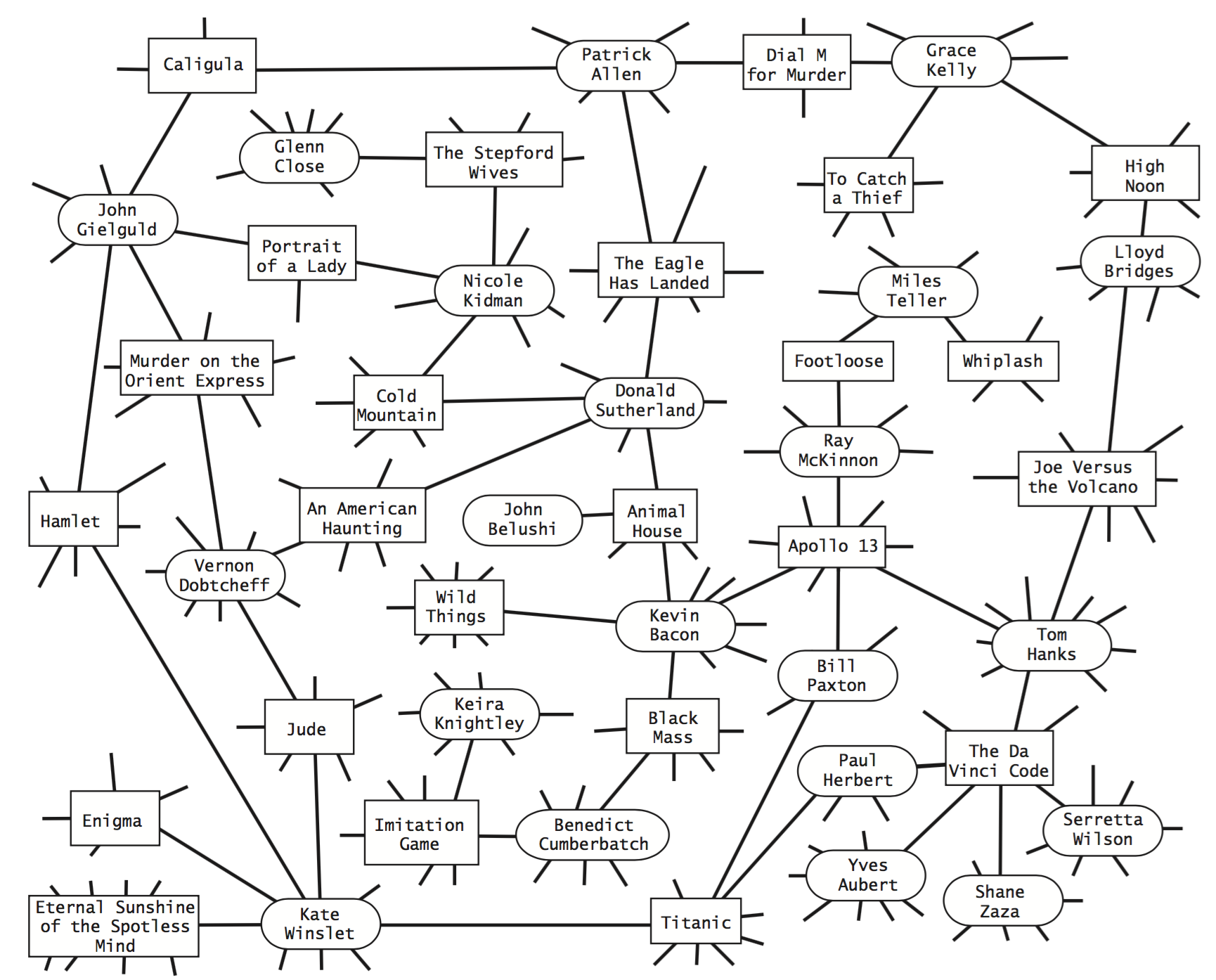We provide a number of sample data files (encoded using UTF-8 for compatibility with In). Each line in a file consists of a movie, followed by a list of performers that appeared in the movie, separated by the / delimiter.

FILE DESCRIPTION MOVIES ACTORS EDGES
cast.06.txt movies release in 2006 8780 84236 103815
cast.00-06.txt movies release since 2000 52195 348497 606531
cast.G.txt movies rated G by MPAA 1288 21177 28485
cast.PG.txt movies rated PG by MPAA 3687 74724 116715
cast.PG13.txt movies rated PG-13 by MPAA 2538 70325 103265
cast.mpaa.txt movies rated by MPAA 21861 280624 627061
cast.action.txt action movies 14938 139861 266485
cast.rated.txt popular movies 4527 122406 217603
cast.all.txt over 250,000 movies 285462 933864 3317266
These input files are as of November 2, 2006. The data is taken from the Internet Movie Database.

Inverted index. Program IndexGraph.java takes a query which is the name of a movie (in which case it prints the list of performers that appear in that movie) or the name of a performer (in which case it prints the list of movies in which that performer has appeared).

## Shortest paths in graphs.

Given two vertices in a graph, a path is a sequence of edges connecting them. A shortest path is one with minimal length over all such paths.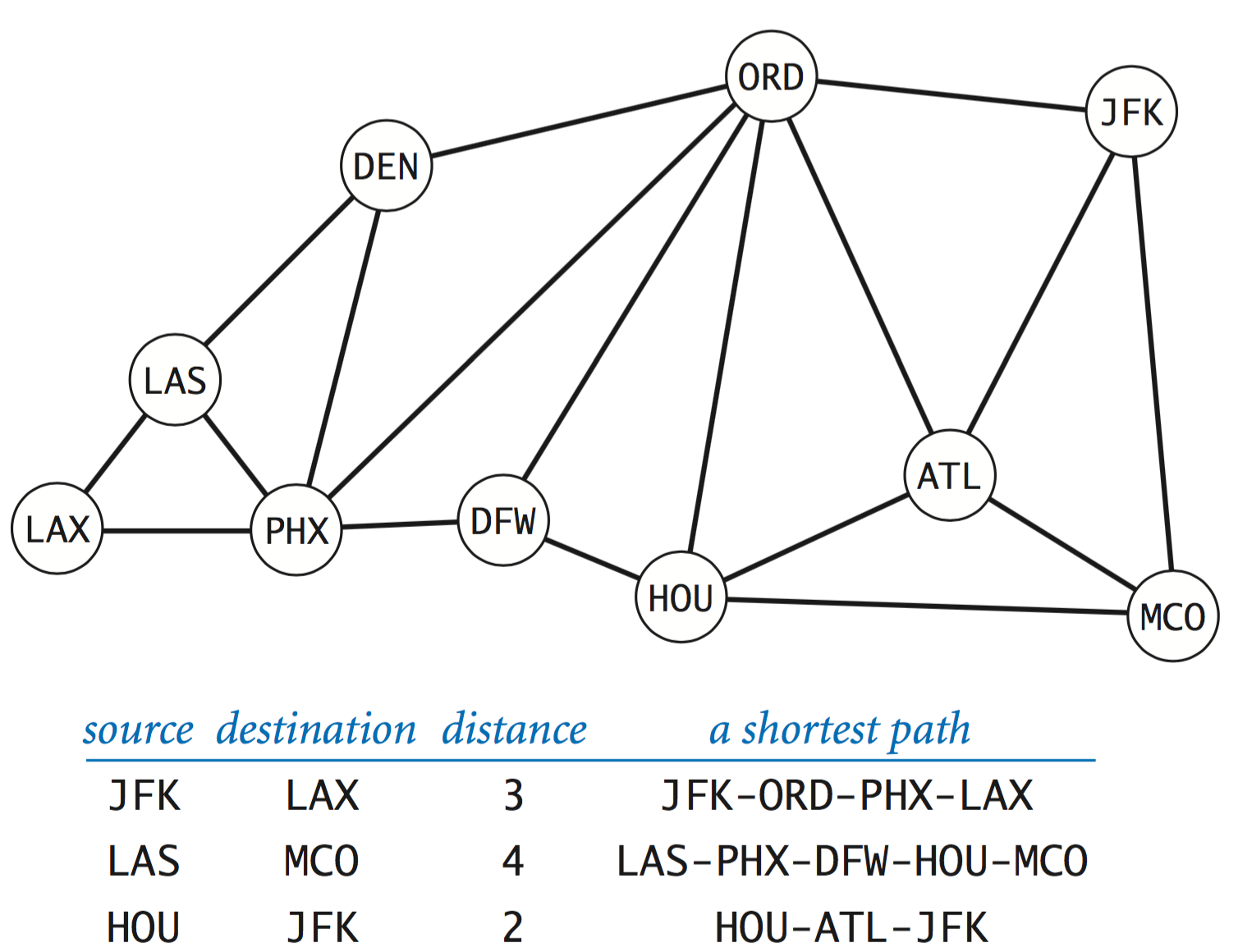PathFinder.java computes the shortest paths in a graph using a classic algorithm known as breadth-first search.

## Degrees of separation.

One of the classic applications of shortest-paths algorithms is to find the degrees of separation of individuals in social networks. To fix ideas, we use the movie–performer. Kevin Bacon is a prolific actor who appeared in many movies. We assign every performer who has appeared in a movie a Bacon number: Bacon himself is 0, any performer who has been in the same cast as Bacon has a Kevin Bacon number of 1, any other performer (except Bacon) who has been in the same cast as a performer whose number is 1 has a Kevin Bacon number of 2, and so forth.

Given the name of a performer, the Kevin Bacon game is to find some alternating sequence of movies and performers that lead back to Kevin Bacon. Remarkably, PathFinder.java provides a solution.

 % java PathFinder movies.txt "/" "Bacon, Kevin" Kidman, Nicole Bacon, Kevin Animal House (1978) Sutherland, Donald (I) Cold Mountain (2003) Kidman, Nicole distance 2 Hanks, Tom Bacon, Kevin Apollo 13 (1995) Hanks, Tom distance 1 
The six degrees of separation rule suggests that most actors will have a Kevin Bacon number of 6 or less. In fact, most have a Kevin Bacon number of 3 or less!

## Small-world graphs.

Scientists have identified a particularly interesting class of graphs, known as small-world graphs, that arise in numerous applications in the natural and social sciences. Small-world graphs are characterized by the following three properties:
• They are sparse: the number of edges is much smaller than the total potential number of edges for a graph with the specified number of vertices.
• They have short average path lengths: if you pick two random vertices, the length of the shortest path between them is short.
• They exhibit local clustering: if two vertices are neighbors of a third vertex, then the two vertices are likely to be neighbors of each other.SmallWorld.java computers the average degree, average path length, and clustering coefficient of a graph.

## Performer–performer graph.

Our movie–performer graph is not a small-world graph, because it is bipartite and therefore has a clustering coefficient of 0. However, the simpler performer–performer graph defined by connecting two performers by an edge if they appeared in the same movie is a classic example of a small-world graph (after discarding performers not connected to Kevin Bacon). The diagram below illustrates the movie–performer and performer–performer graphs associated with a tiny movie-cast file.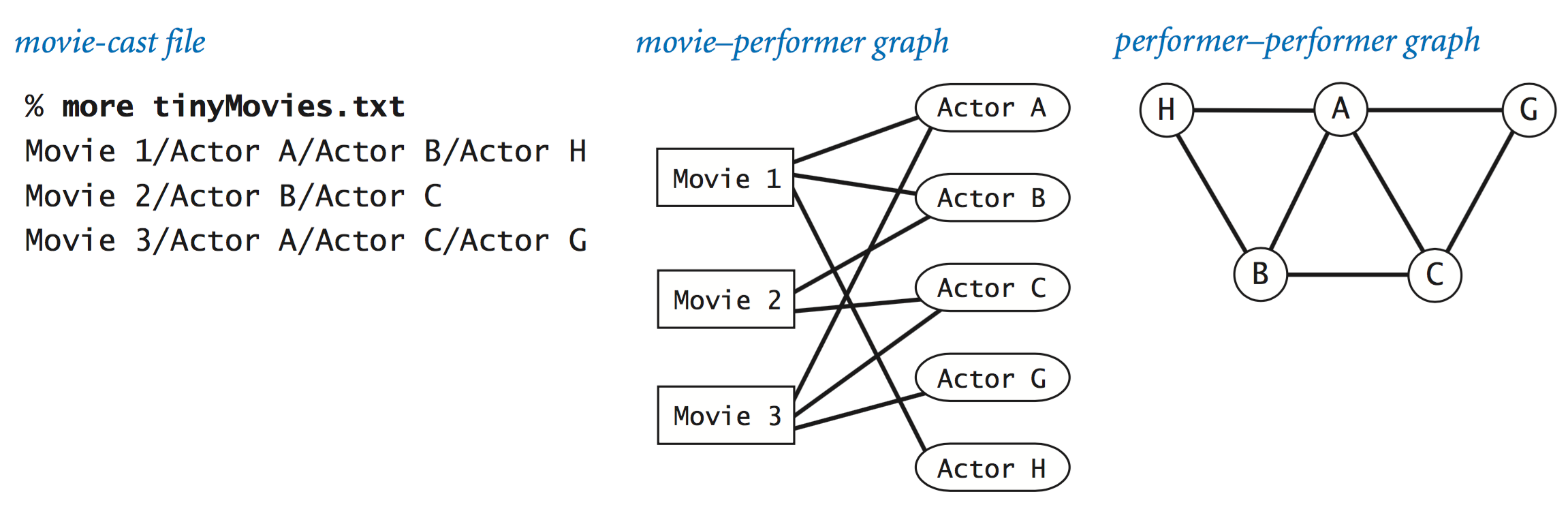Performer.java creates a performer–performer graph from a file in our movie-cast input format. You can use it to verify that the such graphs are small-world graphs.

#### Exercises

1. Add to Graph.java the implementations of V() and E() that return the number of vertices and edges in the graph, respectively.
2. Add to Graph.java a method degree() that takes a string argument and returns the degree of the specified vertex. Use this method to find the performer in the file movies.txt who has appeared in the most movies.
3. Add to Graph.java a method hasVertex() that takes a string argument and returns true if it names a vertex in the graph, and false otherwise.
4. Add to Graph.java a method hasEdge() that takes two string arguments and returns true if they specify an edge in the graph, and false otherwise.
5. True or false: At some point during breadth-first search the queue can contain two vertices, one whose distance from the source is 7 and one whose distance is 9.

Solution: False. The queue can contain vertices of at most two distinct distances d and d + 1. Breadth-first search examines the vertices in increasing order of distance from the source. When examining a vertex at distance d, only vertices of distance d−1 can be enqueued.

6. Suppose you use a stack instead of a queue for breadth-first search in PathFinder.java. Does it still compute a path from the source to each vertex? Does it still computer shortest paths?

Solution: Yes, no.

7. What would be the effect of using a queue instead of a stack when forming the shortest paths in pathTo()?

Solution: It would return the vertices in reverse order.

#### Creative Exercises

1. Histogram. Write a program BaconHistogram.java that prints a histogram of Kevin Bacon numbers, indicating how many performers from movies.txt have a Bacon number of 0, 1, 2, 3, .... Include a category for those who have an infinite number (not connected at all to Kevin Bacon).
  # Freq ------------ 0 1 1 2083 2 187072 3 515582 4 113741 5 8269 6 772 7 93 8 7 Inf 28942 
2. Word ladder. Write a program WordLadder.java that takes two 5-letter strings as command-line arguments,reads in a list of 5-letter words from standard input, and prints out a shortest word ladder using the words on standard input connecting the two strings (if it exists). Two words are adjacent in a word ladder chain if they differ in exactly one letter. As an example, the following word ladder connects green and brown:
 green greet great groat groan grown brown 
This game, originally known as doublet, was invented by Lewis Carroll. You can also try out your program on this list of 6 letter words.
3. All paths. Write a Graph.java client AllPaths.java whose constructor takes a Graph as argument and supports operations to count or print all simple paths between two given vertices s and t in the graph. A simple path is a path that does not repeat any vertices. In two-dimensional grids, such paths are referred to as self-avoiding walksWarning: there might be exponentially paths, so don't run this on large graphs.
4. Directed graphs. Implement a Digraph.java data type that represents directed graph, where the direction of edges is significant: addEdge(v, w) means to add an edge from v to w but not from w to v. Replace adjacentTo() with two methods: one to give the set of vertices having edges directed to them from the argument vertex, and the other to give the set of vertices having edges directed from them to the argument vertex. Explain how PathFinder.java would need to be modified to find shortest paths in directed graphs.

#### Web Exercises

1. All shortest pairs. Write a program AllShortestPaths.java that builds a graph from a file, and reads source-destination requests from standard input and prints a shortest path in the graph from the source to the destination.
2. Faster word ladders. To speed things up (if the word list is very large), don't write a nested loop to try all pairs of words to see if they are adjacent. To handle 5 letter words, first sort the word list. Words that only differ in their last letter will appear consecutively in the sorted list. Sort 4 more times, but cyclically shift the letters one position to the right so that words that differ in the ith letter will appear consecutively in one of the sorted lists.

Try out this approach using a larger word list with words of different sizes. Two words of different lengths are neighbors if the smaller word is the same as the bigger word, minus the last letter, e.g., brow and brown.

3. Checkers. Extend the rules of checkers to an N-by-N checkerboard. Show how to determine whether a checker can become in king in the current move. (Use BFS or DFS.) Show how to determine whether black has a winning move. (Find an Euler path.)
4. Combinational circuits. Determining the truth value of a combinational circuit given its inputs is a graph reachability problem (on a directed acyclic graph).
5. Hex. The game of Hex is played on a trapezoidal grid of hexagons....
• Describe how to detect whether white or black has won the game using either BFS or DFS.
• Prove that the game cannot end in a tie. Hint: consider the set of cells reachable from the left side of the board.
• Prove that the first player in Hex can always win if they play optimally. Hint: if the second player had a winning strategy, you could choose a random cell initially, and then just copy the second player's winning strategy. In game theory, this technique is known as strategy stealing.
6. Write a program MovieStats.java that reads in a movie data set and prints out the number of distinct actors and movies.
7. Modify Bacon.java so that the user types two actors (one per line) and the program prints a shortest chain between the two actors.
8. Modify Graph.java to include a method iterator() that returns an Iterator to the graph's vertices. Also, make Graph implement the Iterable<String> interface so that you can use it with foreach to iterate over the graph's vertices.
 public Iterator iterator() { return st.iterator(); } 
9. Garbage collection. Reference counting vs. mark-and-sweep. Automatic memory management in languages like Java is a challenging problem. Allocating memory is easy, but discovering when a program is finished with memory (and reclaiming it) is more difficult. Reference counting: doesn't work with circular linked structure. Mark-and-sweep algorithm. Root = local variables and static variables. Run DFS from roots, marking all variables references from roots, and so on. Then, make second pass: free all unmarked objects and unmark all marked objects. Or a copying GC would then move all of the marked objects to a single memory area. Uses one extra bit per object. JVM must pause while garbage collection occurs. Fragments memory.
10. Power law of web links. (Micahel Mitzenmacher) The indegrees and outdegrees of World Wide Web obey a power law. Can be modeled by preferred attachment process. Suppose that each web page has exactly one outgoing link. Each page is created one at a time, starting with a single page that points to itself. With probability p < 1, it links to one of the existing pages, chosen uniformly at random. With probability 1-p it links to an existing page with probability proportional to the number of incoming links of that page. This rule reflects the common tendency for new web pages to point to popular pages. Write a program to simulate this process and plot a histogram of the number of incoming links. Answer: you should observe that the fraction of pages with indegree k is proportional to k^(-1 / (1 - p)).